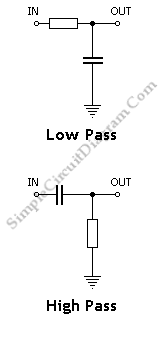# Low-Pass and High-Pass RC Filter Frequency Calculator

This is a simple Low-Pass and High-Pass RC Filter Frequency Calculator. This calculator is used to calculate the corner frequency of low pass and high pass filter. To change from low pass to high pass, just switch the position of the resistor and capacitor. The corner frequency does not change because the calculator assumes a low source impedance. Here is the calculator and the circuit:In the following  calculator , to find the cut-off frequency, input any two values in the form box and hit the calculate button.